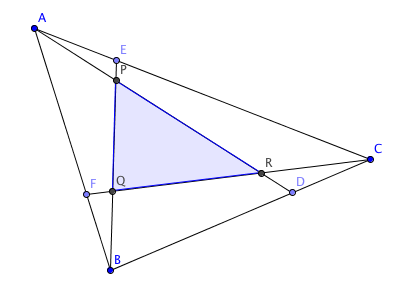# Area Ratio

Geometry Level 5

$ABC$ is a triangle. Points $D, E$ and $F$ are on $BC, CA$ and $AB$, respectively, such that $\frac {BD}{DC} = \frac {CE}{EA} = \frac {AF}{FB} = 2$. Let $AD$ and $BE$ intersect at $P$, $BE$ and $CF$ intersect at $Q$, and $CF$ and $AD$ intersect at $R$. What is the ratio $[ABC]:[PQR]$?Details and assumptions

$[PQRS]$ represents the area of the figure $PQRS$.

×